Скачать презентацию Order Quantities when Demand is Approximately Level Chapter

d89a2a53842882a227d9cafa5f6c0631.ppt

• Количество слайдов: 42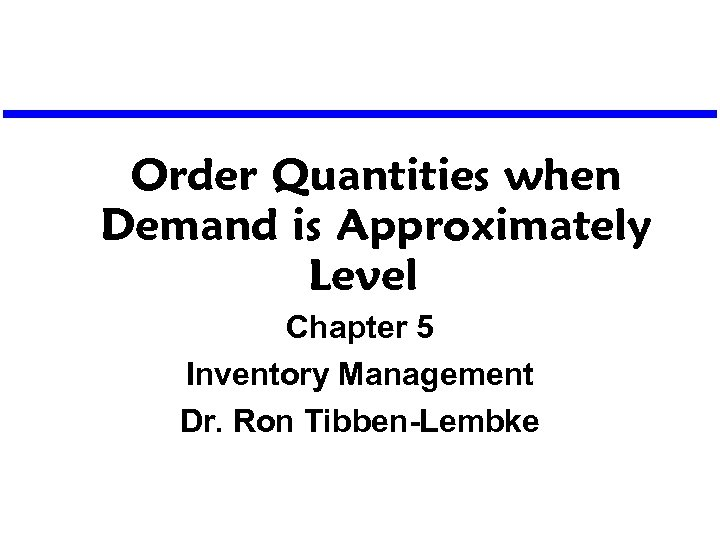Order Quantities when Demand is Approximately Level Chapter 5 Inventory Management Dr. Ron Tibben-Lembke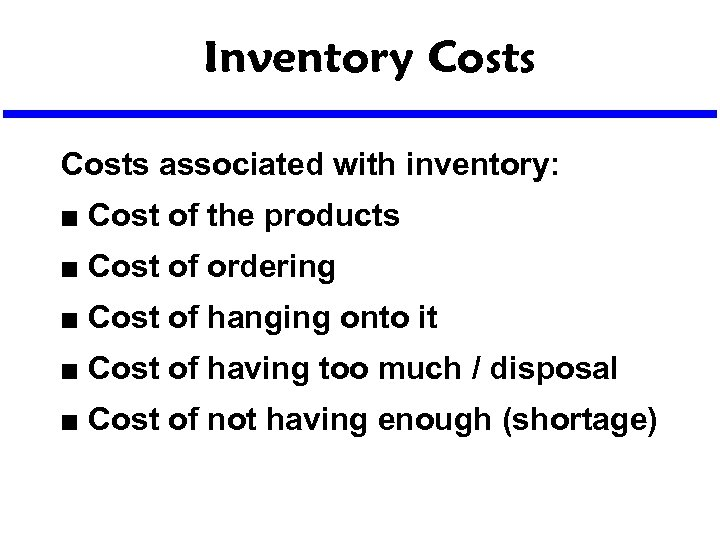Inventory Costs associated with inventory: n Cost of the products n Cost of ordering n Cost of hanging onto it n Cost of having too much / disposal n Cost of not having enough (shortage)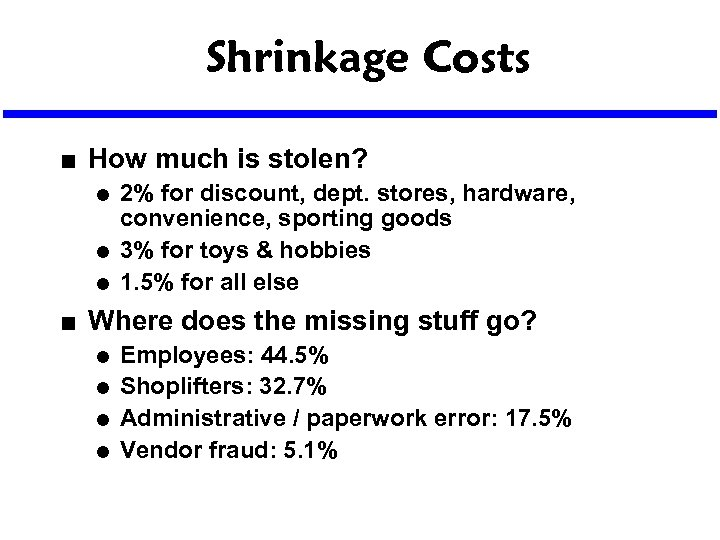Shrinkage Costs n How much is stolen? l l l n 2% for discount, dept. stores, hardware, convenience, sporting goods 3% for toys & hobbies 1. 5% for all else Where does the missing stuff go? l l Employees: 44. 5% Shoplifters: 32. 7% Administrative / paperwork error: 17. 5% Vendor fraud: 5. 1%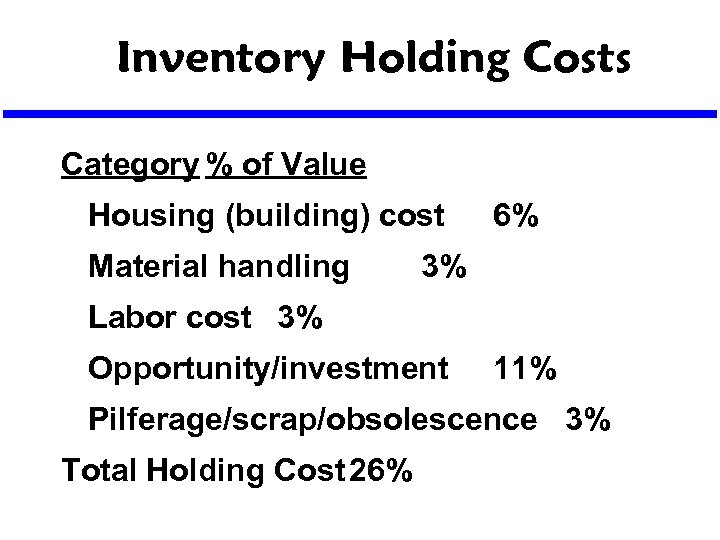Inventory Holding Costs Category % of Value Housing (building) cost Material handling 6% 3% Labor cost 3% Opportunity/investment 11% Pilferage/scrap/obsolescence 3% Total Holding Cost 26%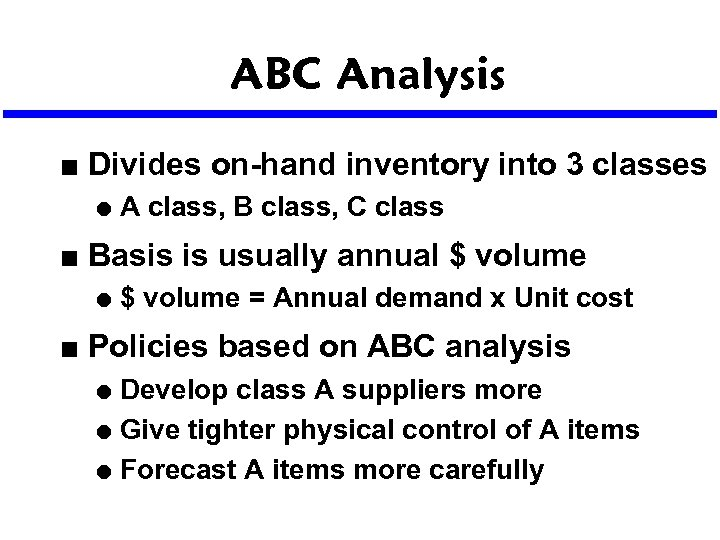ABC Analysis n Divides on-hand inventory into 3 classes l n Basis is usually annual \$ volume l n A class, B class, C class \$ volume = Annual demand x Unit cost Policies based on ABC analysis Develop class A suppliers more l Give tighter physical control of A items l Forecast A items more carefully l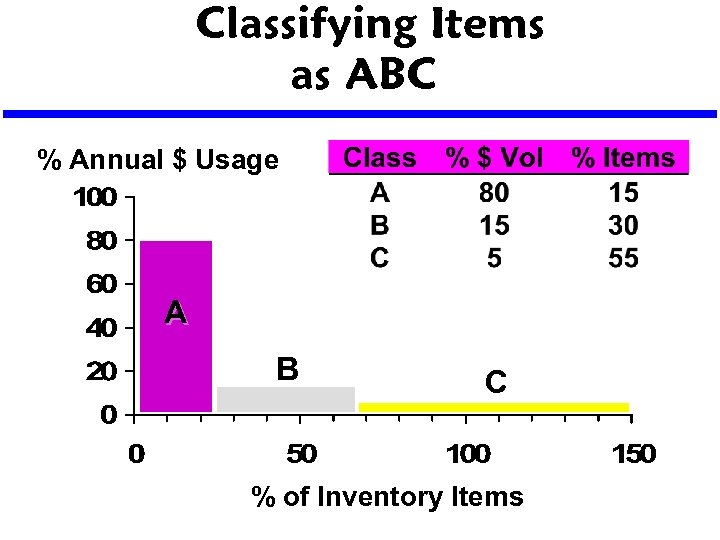Classifying Items as ABC % Annual \$ Usage A B C % of Inventory Items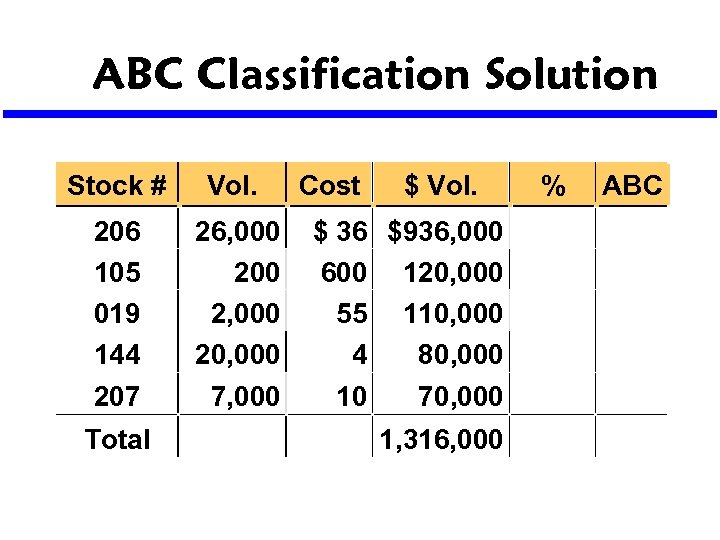ABC Classification Solution Stock # Vol. 206 105 019 144 207 26, 000 2, 000 20, 000 7, 000 Total Cost \$ Vol. \$ 36 \$936, 000 600 120, 000 55 110, 000 4 80, 000 10 70, 000 1, 316, 000 % ABC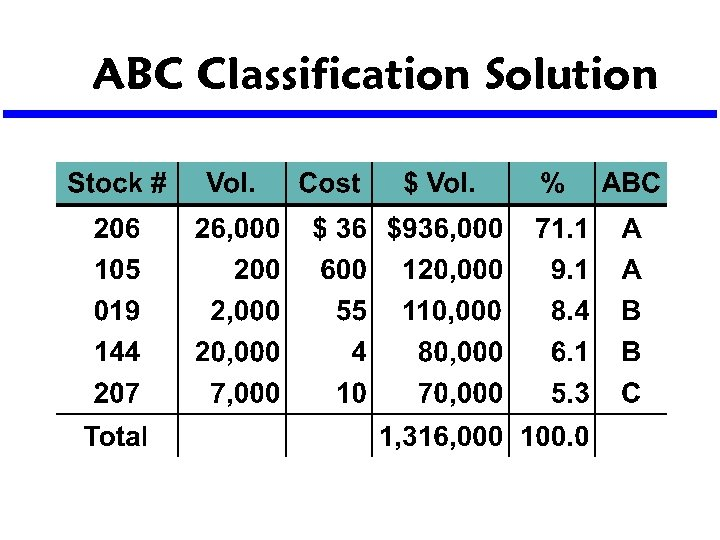ABC Classification Solution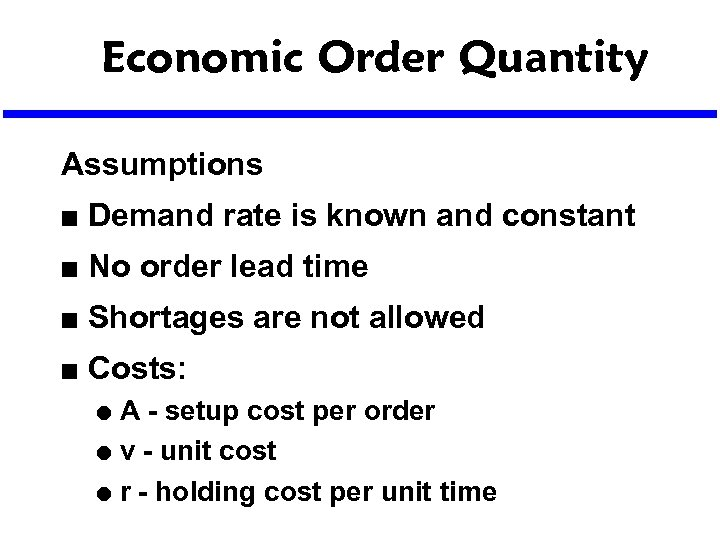Economic Order Quantity Assumptions n Demand rate is known and constant n No order lead time n Shortages are not allowed n Costs: A - setup cost per order l v - unit cost l r - holding cost per unit time l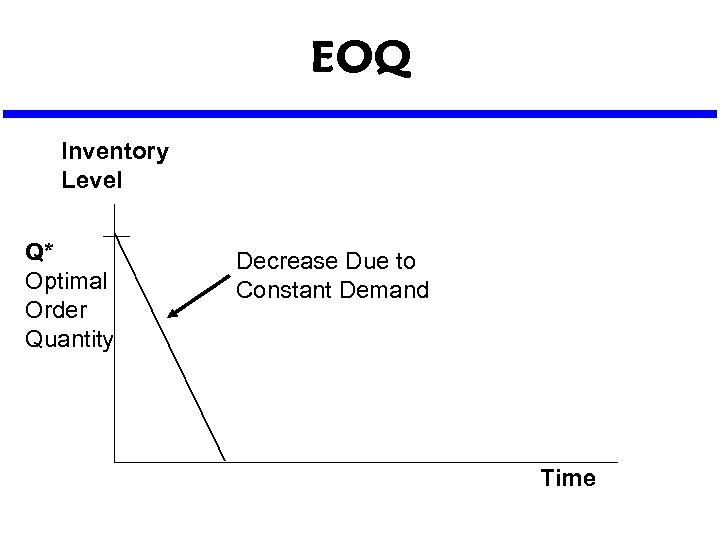EOQ Inventory Level Q* Optimal Order Quantity Decrease Due to Constant Demand Time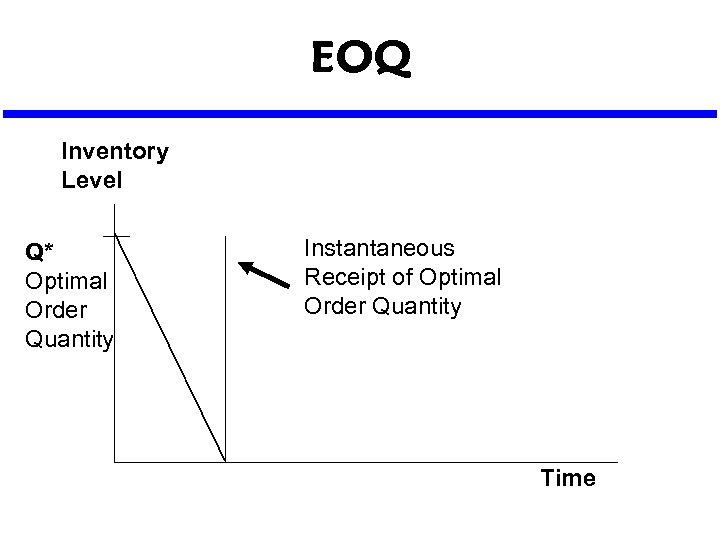EOQ Inventory Level Q* Optimal Order Quantity Instantaneous Receipt of Optimal Order Quantity Time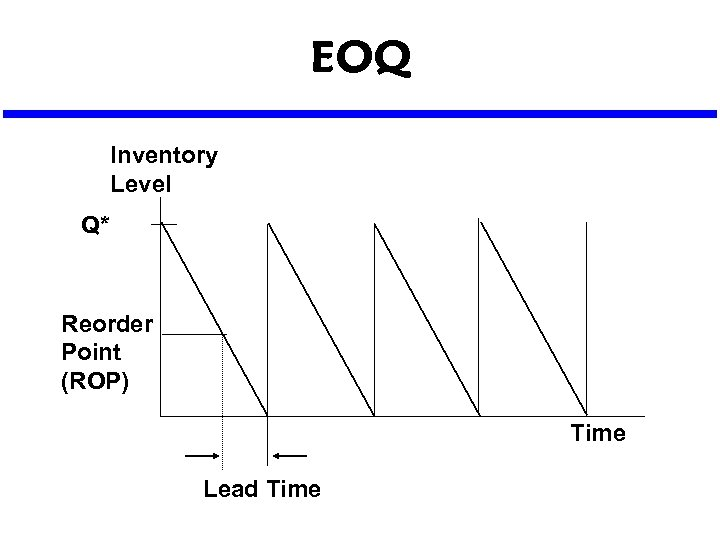EOQ Inventory Level Q* Reorder Point (ROP) Time Lead Time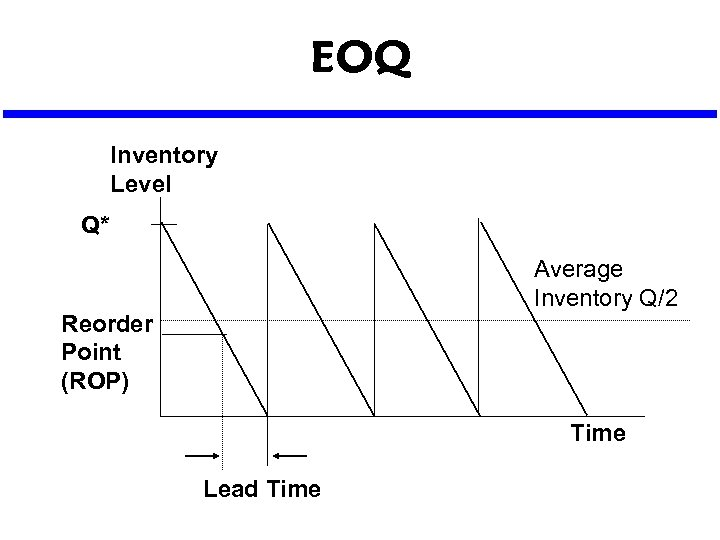EOQ Inventory Level Q* Average Inventory Q/2 Reorder Point (ROP) Time Lead Time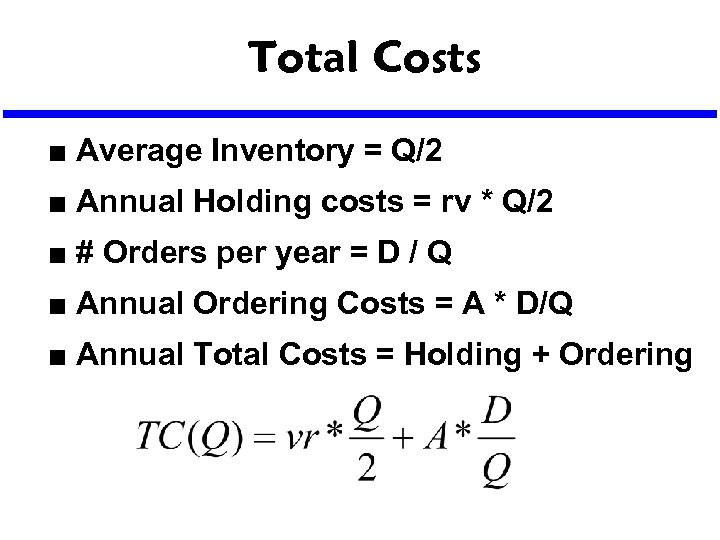Total Costs n Average Inventory = Q/2 n Annual Holding costs = rv * Q/2 n # Orders per year = D / Q n Annual Ordering Costs = A * D/Q n Annual Total Costs = Holding + Ordering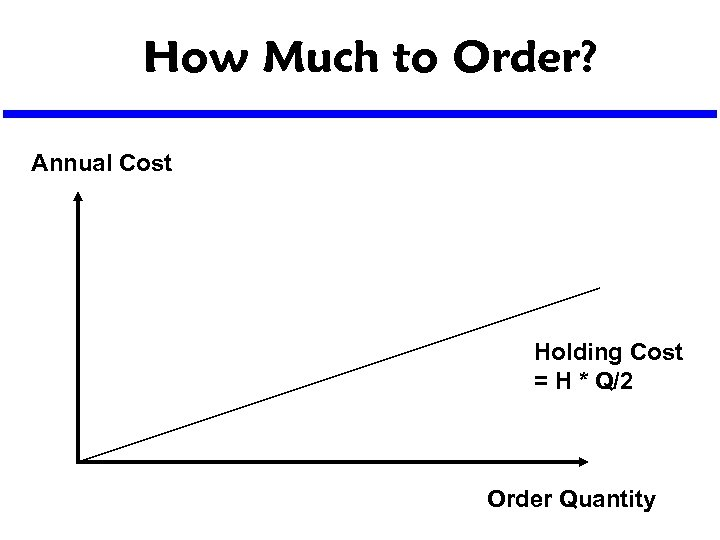How Much to Order? Annual Cost Holding Cost = H * Q/2 Order Quantity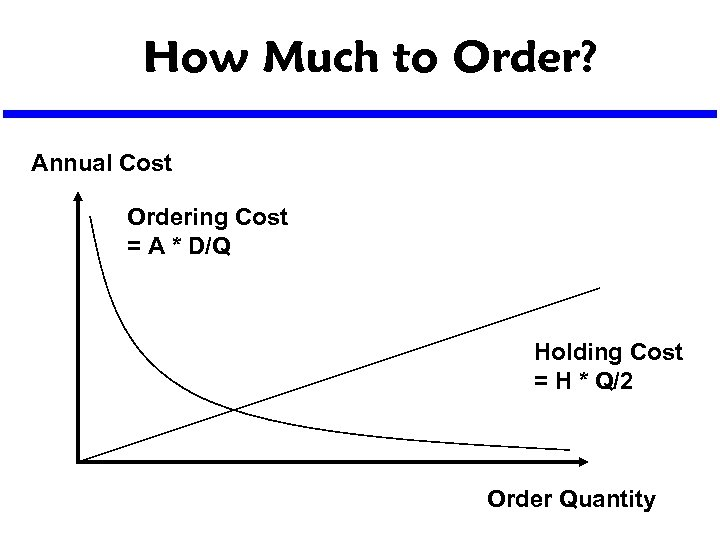How Much to Order? Annual Cost Ordering Cost = A * D/Q Holding Cost = H * Q/2 Order Quantity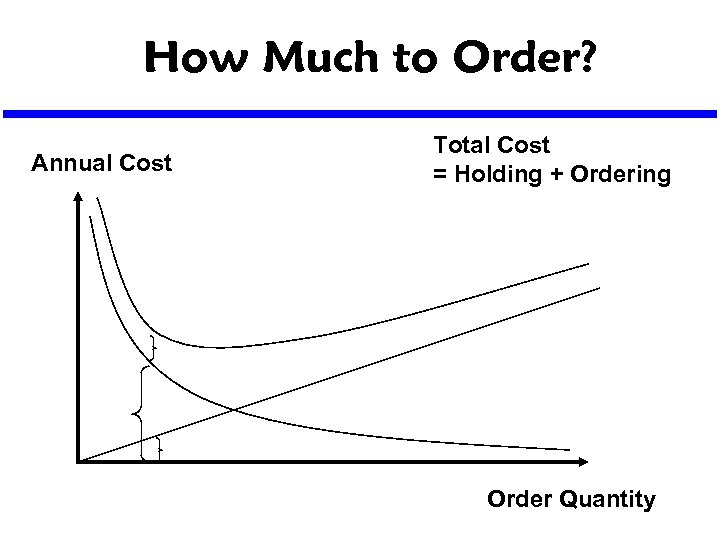How Much to Order? Annual Cost Total Cost = Holding + Ordering Order Quantity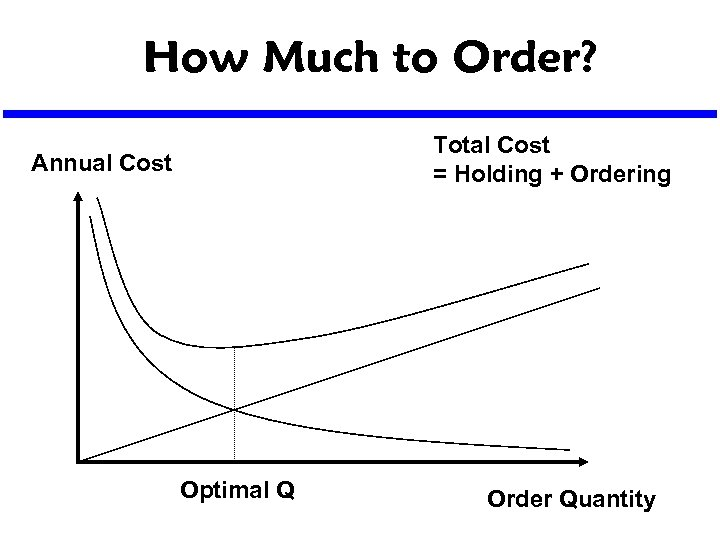How Much to Order? Total Cost = Holding + Ordering Annual Cost Optimal Q Order Quantity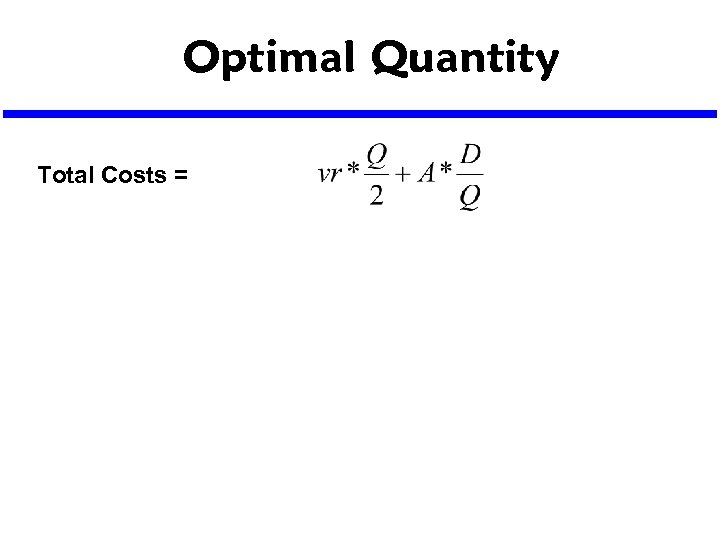Optimal Quantity Total Costs =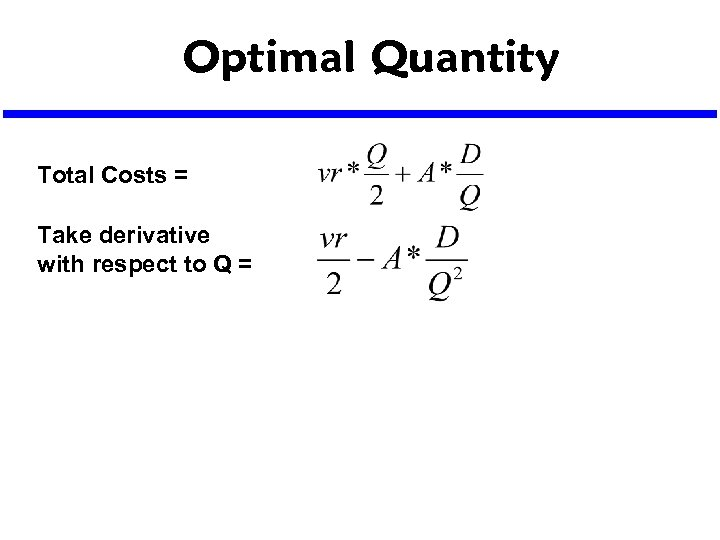Optimal Quantity Total Costs = Take derivative with respect to Q =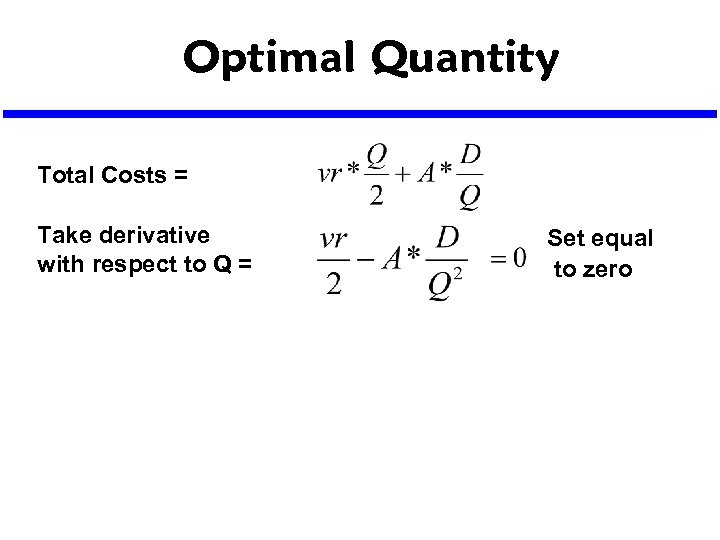Optimal Quantity Total Costs = Take derivative with respect to Q = Set equal to zero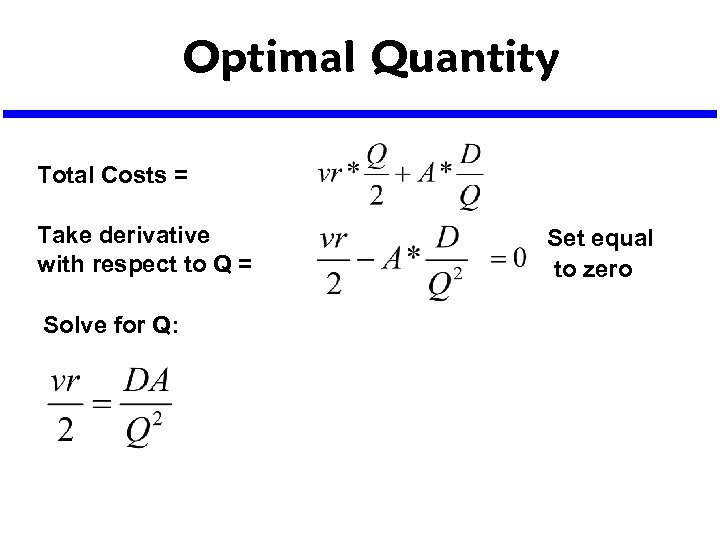Optimal Quantity Total Costs = Take derivative with respect to Q = Solve for Q: Set equal to zero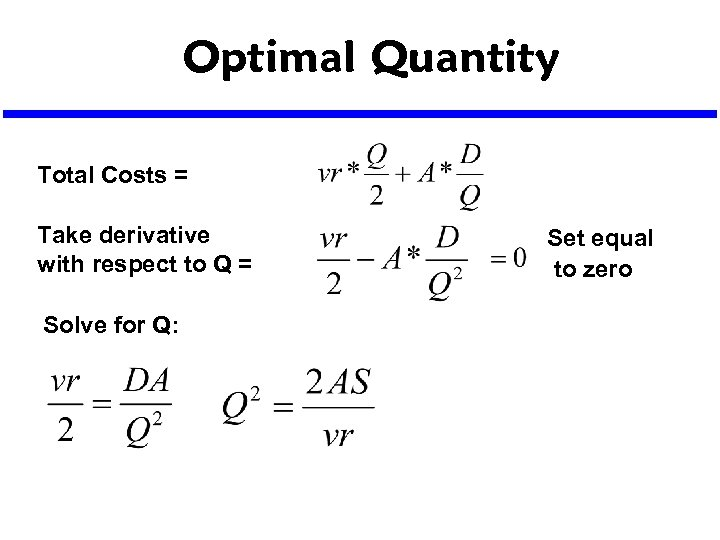Optimal Quantity Total Costs = Take derivative with respect to Q = Solve for Q: Set equal to zero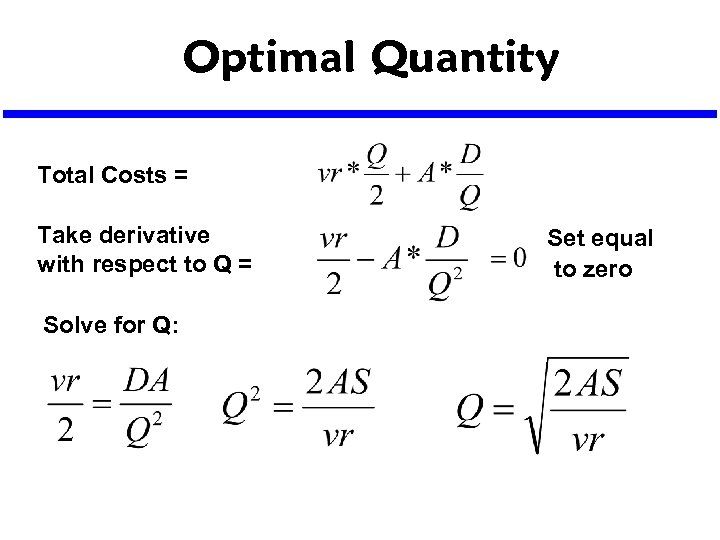Optimal Quantity Total Costs = Take derivative with respect to Q = Solve for Q: Set equal to zero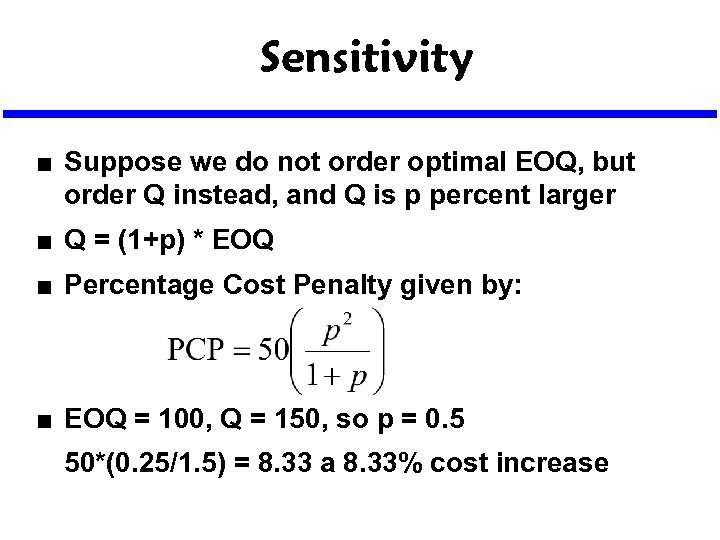Sensitivity n Suppose we do not order optimal EOQ, but order Q instead, and Q is p percent larger n Q = (1+p) * EOQ n Percentage Cost Penalty given by: n EOQ = 100, Q = 150, so p = 0. 5 50*(0. 25/1. 5) = 8. 33 a 8. 33% cost increase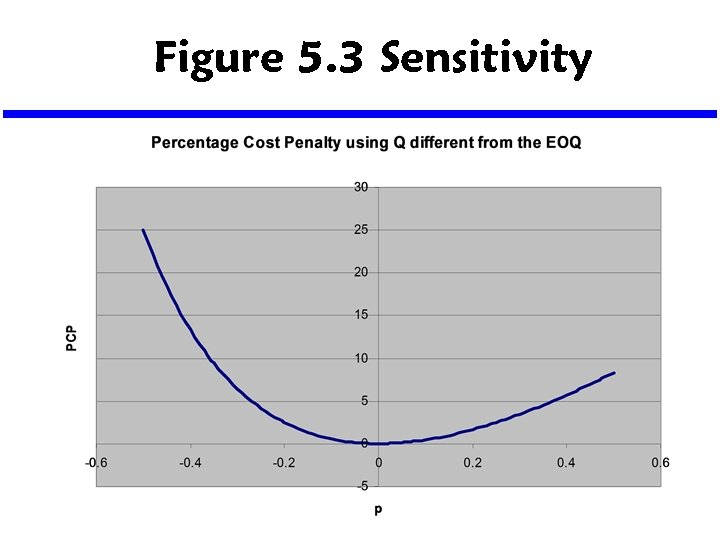Figure 5. 3 Sensitivity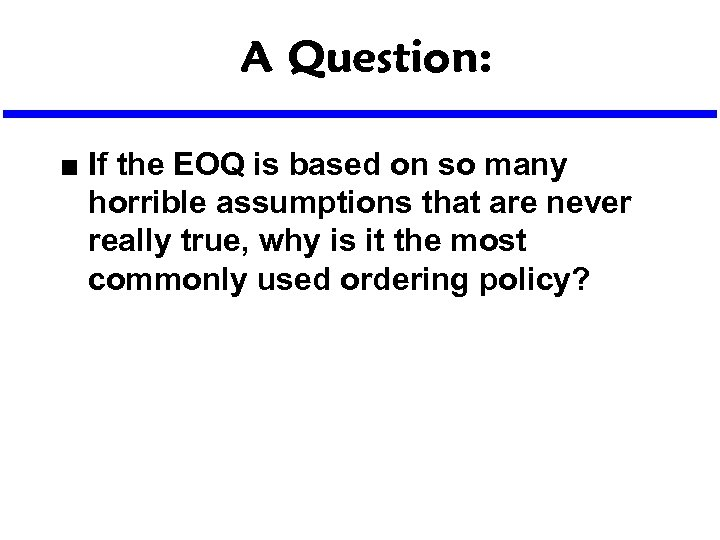A Question: n If the EOQ is based on so many horrible assumptions that are never really true, why is it the most commonly used ordering policy?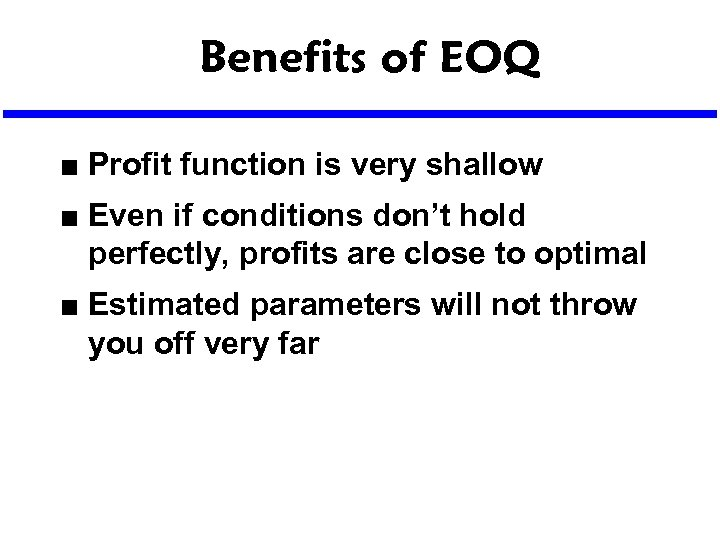Benefits of EOQ n n n Profit function is very shallow Even if conditions don’t hold perfectly, profits are close to optimal Estimated parameters will not throw you off very far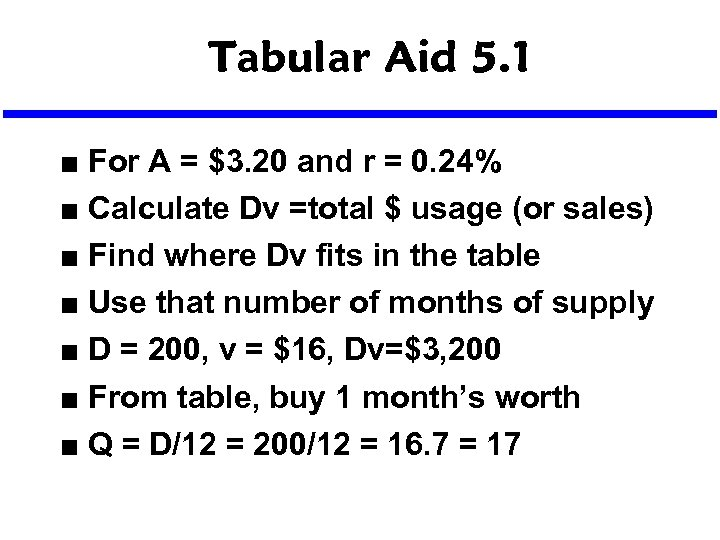Tabular Aid 5. 1 n n n n For A = \$3. 20 and r = 0. 24% Calculate Dv =total \$ usage (or sales) Find where Dv fits in the table Use that number of months of supply D = 200, v = \$16, Dv=\$3, 200 From table, buy 1 month’s worth Q = D/12 = 200/12 = 16. 7 = 17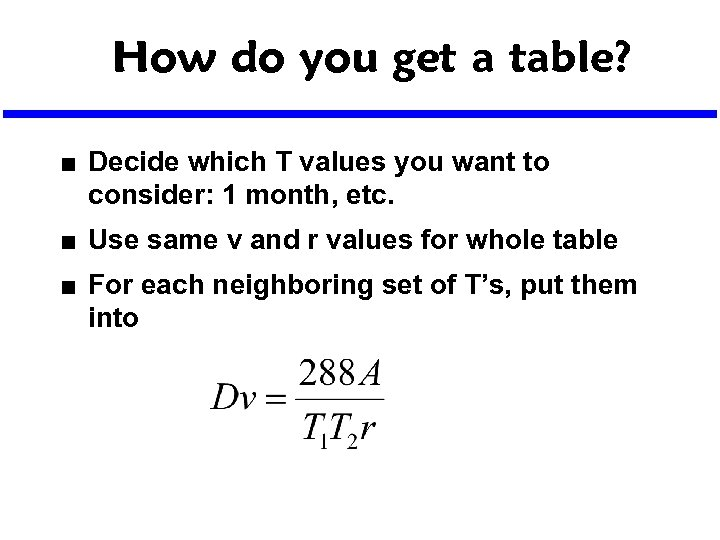How do you get a table? n n n Decide which T values you want to consider: 1 month, etc. Use same v and r values for whole table For each neighboring set of T’s, put them into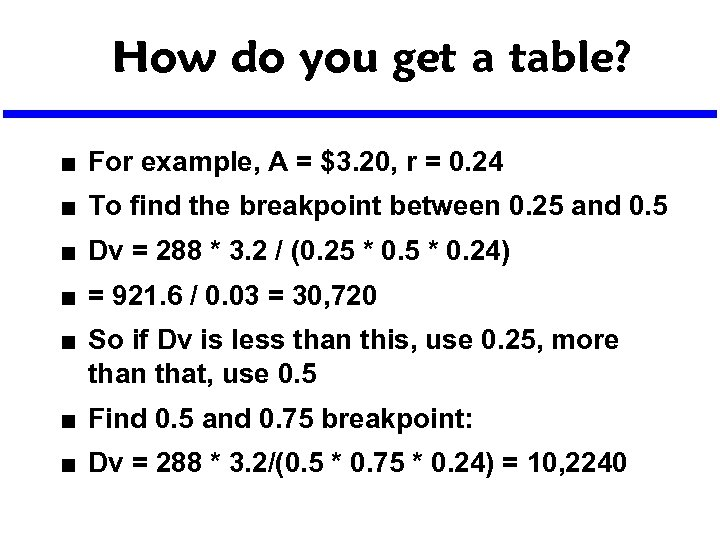How do you get a table? n For example, A = \$3. 20, r = 0. 24 n To find the breakpoint between 0. 25 and 0. 5 n Dv = 288 * 3. 2 / (0. 25 * 0. 24) n = 921. 6 / 0. 03 = 30, 720 n So if Dv is less than this, use 0. 25, more than that, use 0. 5 n Find 0. 5 and 0. 75 breakpoint: n Dv = 288 * 3. 2/(0. 5 * 0. 75 * 0. 24) = 10, 2240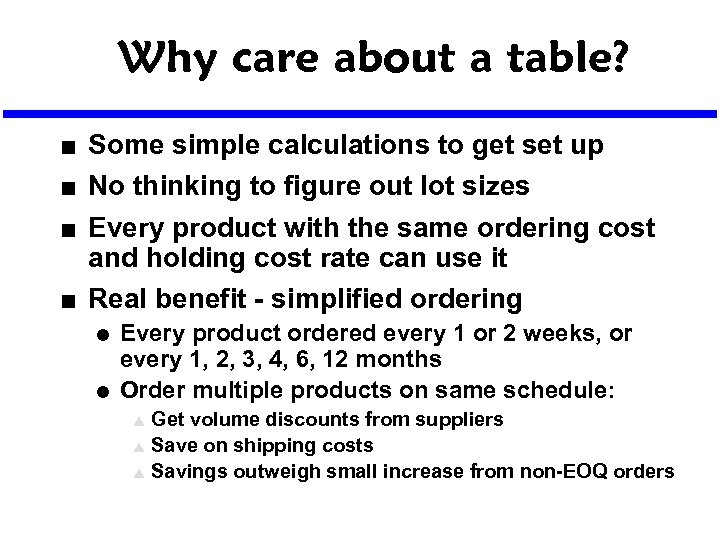Why care about a table? n n Some simple calculations to get set up No thinking to figure out lot sizes Every product with the same ordering cost and holding cost rate can use it Real benefit - simplified ordering l l Every product ordered every 1 or 2 weeks, or every 1, 2, 3, 4, 6, 12 months Order multiple products on same schedule: Get volume discounts from suppliers s Save on shipping costs s Savings outweigh small increase from non-EOQ orders s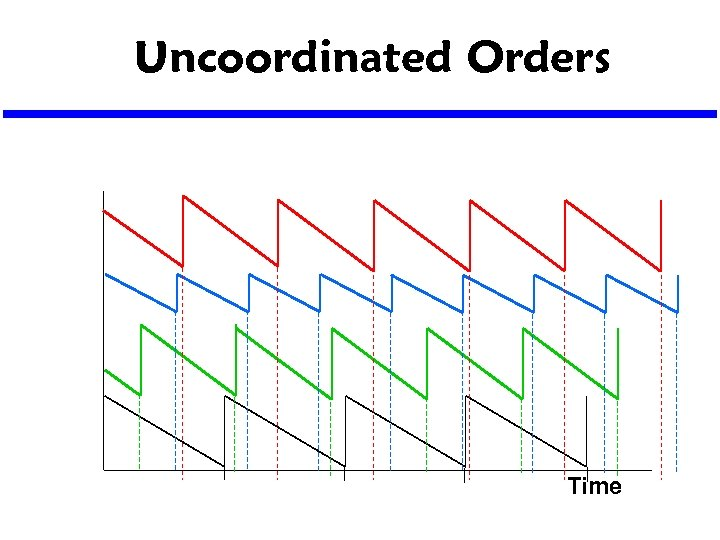Uncoordinated Orders Time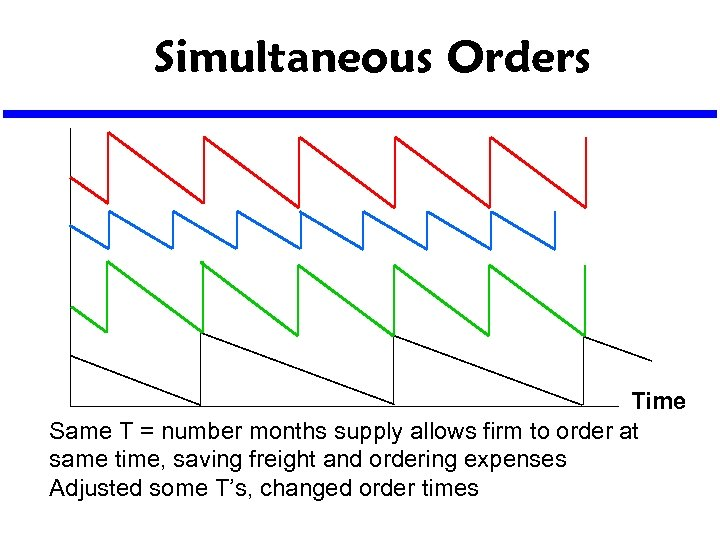Simultaneous Orders Time Same T = number months supply allows firm to order at same time, saving freight and ordering expenses Adjusted some T’s, changed order times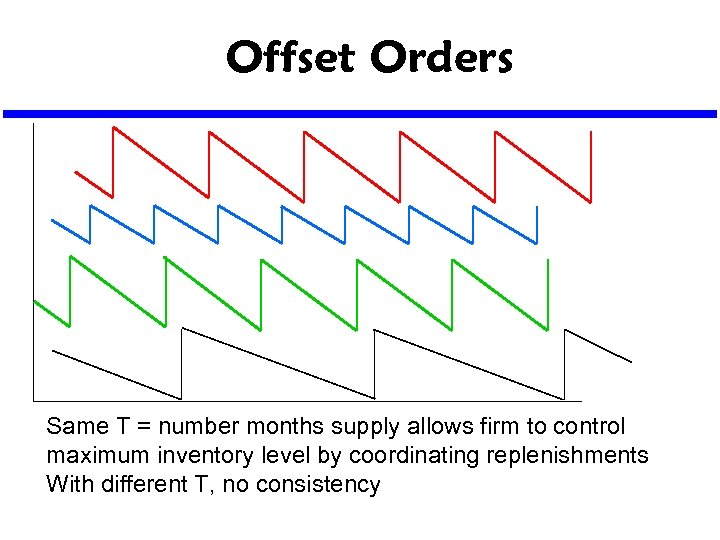Offset Orders Same T = number months supply allows firm to control maximum inventory level by coordinating replenishments With different T, no consistency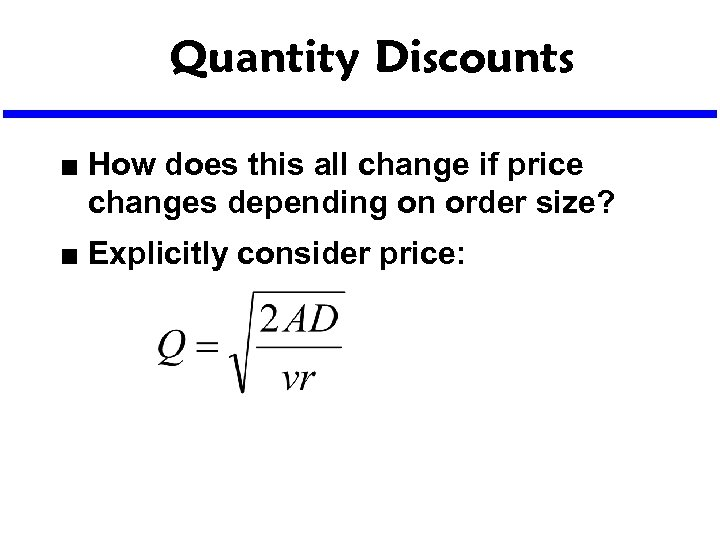Quantity Discounts n n How does this all change if price changes depending on order size? Explicitly consider price: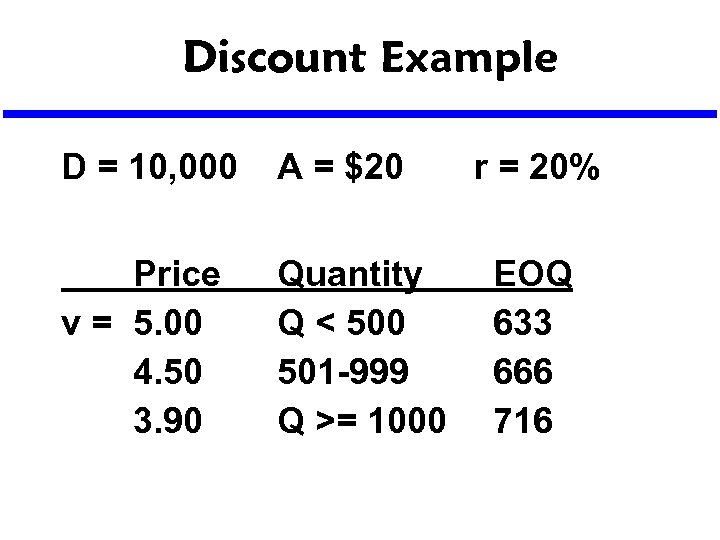Discount Example D = 10, 000 A = \$20 Price v = 5. 00 4. 50 3. 90 Quantity Q < 500 501 -999 Q >= 1000 r = 20% EOQ 633 666 716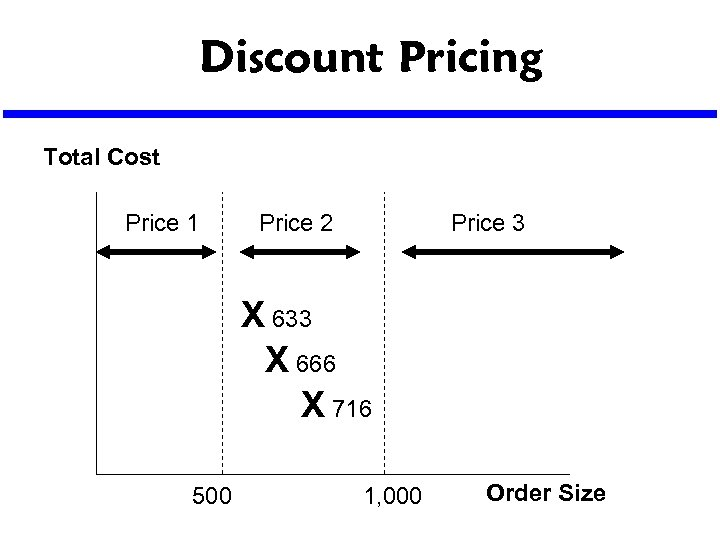Discount Pricing Total Cost Price 1 Price 2 Price 3 X 633 X 666 X 716 500 1, 000 Order Size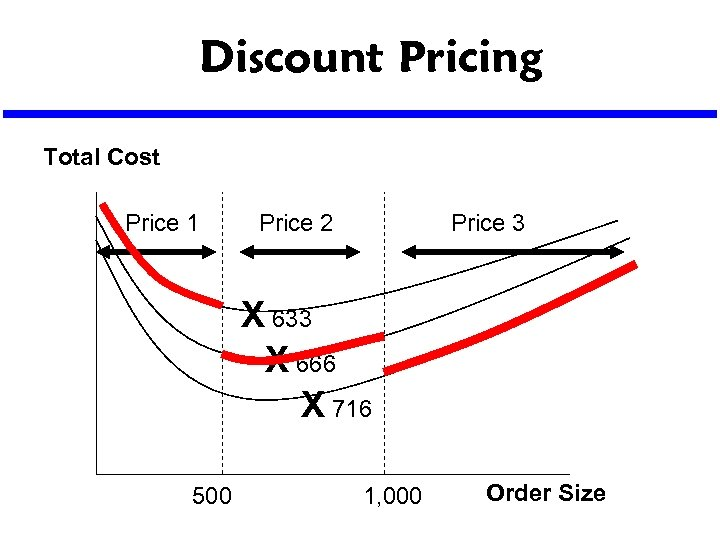Discount Pricing Total Cost Price 1 Price 2 Price 3 X 633 X 666 X 716 500 1, 000 Order Size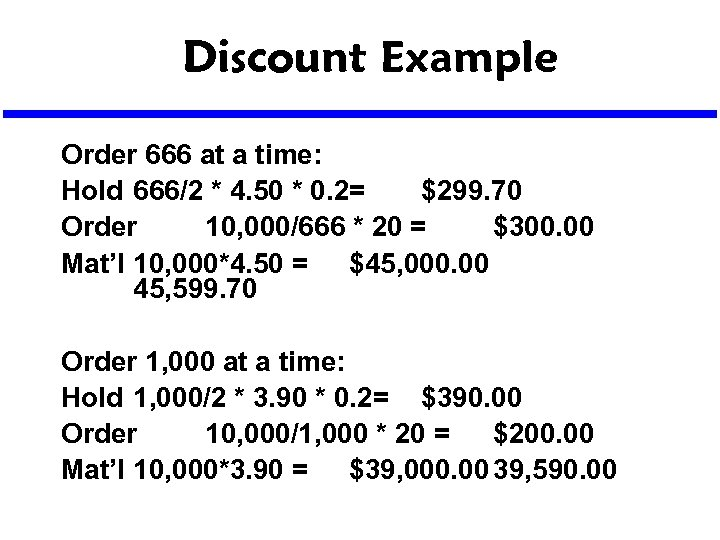Discount Example Order 666 at a time: Hold 666/2 * 4. 50 * 0. 2= \$299. 70 Order 10, 000/666 * 20 = \$300. 00 Mat’l 10, 000*4. 50 = \$45, 000. 00 45, 599. 70 Order 1, 000 at a time: Hold 1, 000/2 * 3. 90 * 0. 2= \$390. 00 Order 10, 000/1, 000 * 20 = \$200. 00 Mat’l 10, 000*3. 90 = \$39, 000. 00 39, 590. 00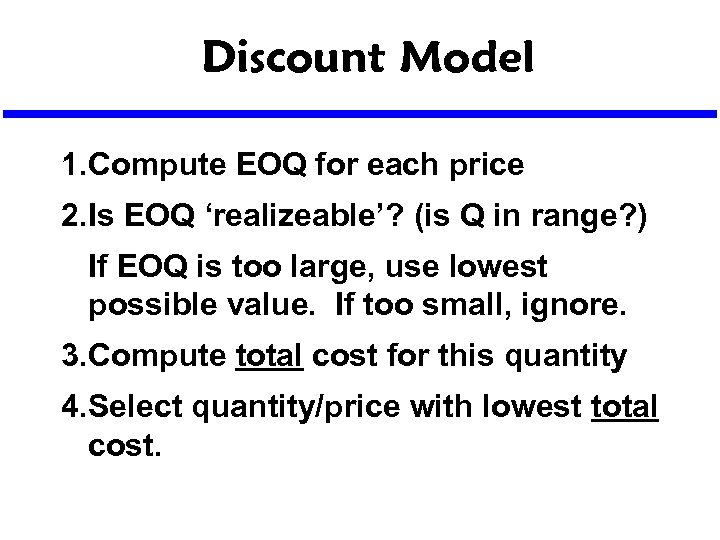Discount Model 1. Compute EOQ for each price 2. Is EOQ ‘realizeable’? (is Q in range? ) If EOQ is too large, use lowest possible value. If too small, ignore. 3. Compute total cost for this quantity 4. Select quantity/price with lowest total cost.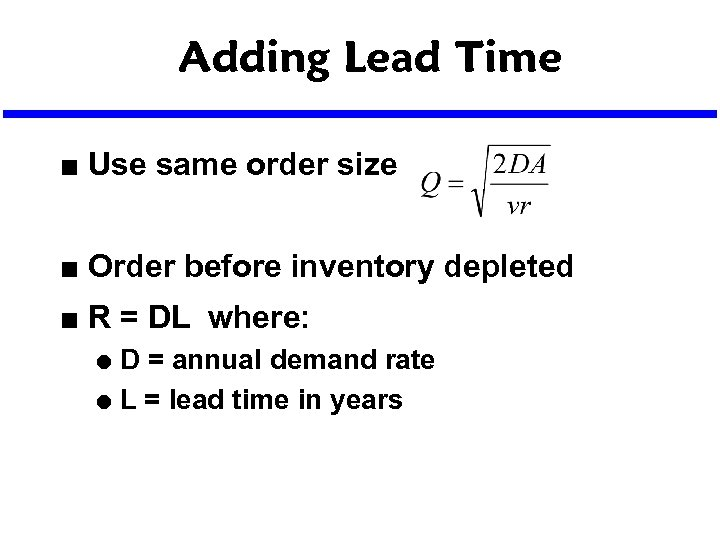Adding Lead Time n Use same order size n Order before inventory depleted n R = DL where: D = annual demand rate l L = lead time in years l